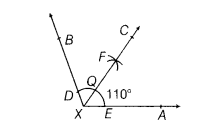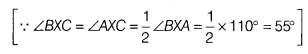# Draw an angle of 110° with the help of a protractor and bisect it. Measure each angle

Draw an angle of 110° with the help of a protractor and bisect it. Measure each angle.

(i) First, draw ∠BXA = 110° with the help of protractor.
(ii) Taking X as centre and with any radius, draw an arc to intersect the rays XA and XB at E and D, respectively.
(iii) Taking D and £ as centre and with the radius greater than 1/2 DE, draw arcs to intersect each other, say at F.(iv) Draw the ray XC passing through F, which is the required bisector of the∠BXA.
On measuring each angle, we get
∠BXC =∠AXC = 55°